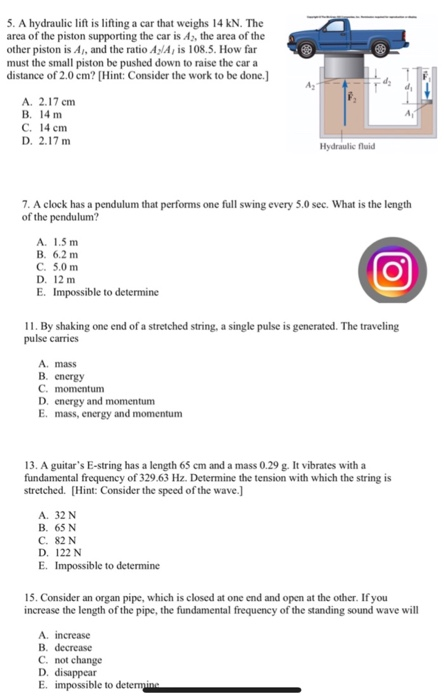# S.A hydraulic lift is lifting a car that weighs 14 kN. The area of the piston...

###### Question:S.A hydraulic lift is lifting a car that weighs 14 kN. The area of the piston supporting the car is A, the area of the other piston is A, and the ratio A/A, is 108.5. How far must the small piston be pushed down to raise the car a distance of 2.0 em? [Hint: Consider the work to be done.] A. 2.17 cm B. 14 m A. C. 14 cm D. 2.17 m Hydraulic fluid 7. A clock has a pendulum that performs one full swing every 5.0 sec. What is the length of the pendulum? A. 1.5 m B. 6.2 m C. 5.0 m D. 12 m E. Impossible to determine 11. By shaking one end of a stretched string, a single pulse is generated. The traveling pulse carries A. mass B. energy C. momentum D. energy and momentum E. mass, energy and momentum 13. A guitar's E-string has a length 65 cm and a mass 0.29 g. It vibrates with a fundamental frequency of 329.63 Hz. Determine the tension with which the string is stretched. [Hint: Consider the speed of the wave.] A. 32 N B. 65 N C. 82 N D. 122 N E. Impossible to determine 15. Consider an organ pipe, which is closed at one end and open at the other. If you increase the length of the pipe, the fundamental frequency of the standing sound wave will A. increase B. decrease C. not change D. disappear E. impossible to determine